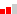# Singapore Mathematics Grade 4 Decimals

## Singapore Mathematics Grade 4 Decimals

(0 Rating) 1 studentsFree

#### Highlights

• Over 5 hours of instruction
• Excellent Instructors
• Convenient: Learn Anywhere!
Certificate

No

Level

IntermediateLanguage

English (US)

# Singapore Mathematics Grade 4: DECIMALS

## Contents:

- Understanding Tenths, Hundredths, and Thousandths

- Fractions and Decimals (Expressing Decimals as Fractions and Fractions as Decimals)

- Comparing an Ordering Decimals

- Number patterns

- Rounding Decimals

- Adding Decimals

- Subtracting Decimals

- Multiplying Decimals by a 1-digit whole number

- Dividing Decimals by a 1-digit whole number

- Word Problems

## Who can join:

- If you are using the Singapore Mathematics Curriculum and would like enrichment and supplemental material.

- If you would like to learn math using the Singapore Mathematics strategies.

## Singapore Mathematics Curriculum:

Math in Focus: Chapter 7/8

Math in Focus 2020: Chapter 4

Primary Mathematics US Ed.: Chapter 8/9

Dimensions Math: Chapter 12/13/1

• 1
Private
• 2
Private

### About Instructor

Singapore Math Learning Center

At Singapore Math Learning Center, we provide online tutoring and online courses to those who are using any Singapore Math curriculums or who are interested in the Singapore Math method. For a free math assessment, please contact us.

click here